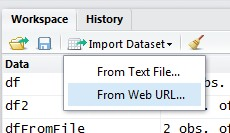# R - Class

A class is just an attribute of an object. You can remove the attribute with the function unclass. The class with then become the type

A list can have different class.

## Management

### Unclass

unclass returns (a copy of) its argument with its class attribute removed. (It is not allowed for objects which cannot be copied, namely environments and external pointers.)

Example: A data frame will return a list:

````class(USArrests)`
```
`````` "data.frame"
```
```

If you unclass it, you will get a list

````class(unclass(USArrests))`
```
`````` "list"
```
```

### Change

Objects can change of class (coercion) using the as.* functions (if available).

• expression
• ….

## Hierarchy

The following hierarchy (order of precedence) occurs when creating an object with several classes:

1. NULL
2. raw
3. numeric (double)
4. expression
5. function

? And:

## Example

See R - List

### Function

````class(mean)`
```
`````` "function"
```
```
````> class(plot)`
```
`````` "function"
```
```

Recommended PagesORE - Install 1.3.1

grant rqrole to grant execute on rqsys.rqGroupEvalImpl for each user. Create all the synonyms listed in rquser.sql for each user. startup scriptfunctions ore.attach(USER, SID, host, password) establishes...R - (Fitting) Linear Model (lm)

lm is a class that contains fitting function.R - (Object|Variable|Symbol)

R provides a number of specialized objects. They are created (instantiated), used and referenced through variable (known as symbol). When you read the term object in the documentation, you can interchange...R - Array

An array is an array of n dimensions with the same class (data type)R - Attribute of an object

where:R - Condition

A condition is a length-one logical vector (FALSE of TRUE) that is not NA. Conditions are always evaluated from left to right.R - Data frame Object

A data frame is a logical implementation of a table in a relational database A data frame inherits all the property and function of an object. It has a list of variables of the same number of rows with...R - Factor (Category, Enumerated Type)

The factor function is used to encode a vector as a factor (ie categorical data). When used with a numeric or a date, a binning function will return a factor. From numeric to a category (For instance,...R - Function

Functions are stored as R objects with the class function Functions can be: used as arguments of other functions nested where: arglist is a list of argument including the three dot argument....R - Inf (Infinity Constant)

Inf represents the infi nity class